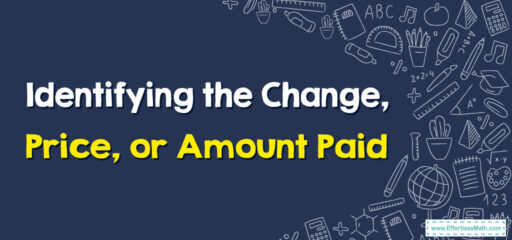# How to Identify the Change, Price, or Amount Paid

Hello young mathematicians! Have you ever wondered how to figure out the cost of your favorite candy, how much money you've spent, or the change you get back when you pay with a bigger note or coin? Well, today, we are embarking on a fun-filled math adventure that will make these puzzles easy as pie. Let's get started, shall we?"Let’s explain these concepts in a way an elementary student can understand. Let’s start with each term:

1. Price: The price is how much something costs. If you want to buy a toy and the tag on it says $15, that means the price of the toy is$15. That’s how much money you need to give to the cashier to get the toy.

2. Amount Paid: The amount paid is the total amount of money you give to the cashier when you buy something. Sometimes, the amount paid is exactly the same as the price.
But let’s say you’re buying a toy that costs $15, and you only have a$20 bill. You give the $20 bill to the cashier. The$20 you give is the amount paid.

3. Change: Change is the money you get back from the cashier when the amount paid is more than the price. If the toy costs $15 and you give the cashier a$20 bill, the cashier will give you some money back.
In this case, the cashier will give you $5 back ($20 – $15 =$5). This $5 is your change. ## A Step-by-step Guide to Identifying the Change, Price, or Amount Paid Here is a simple step-by-step guide that can help a 4th grader understand how to identify the price, amount paid, and change: ### Step 1: Identify the Price The price is the amount of money listed on the item you want to buy. • Look at the price tag or sticker on the item or the amount listed in a menu or catalog. • Sometimes, you might be buying more than one item, so you need to add up the prices of all the items to find the total price. ### Step 2: Identify the Amount Paid The amount paid is the total money you give to the cashier when you are ready to pay for your items. • This could be the exact amount of the total price if you have the exact money. • If you don’t have the exact money, you might give more money than the total price, expecting to receive change. ### Step 3: Calculate the Change Change is the money you receive back when the amount paid is more than the price. • If you paid more than the total price, the cashier should give you a change. • To calculate the change, subtract the total price from the amount paid. ## Related to This Article ### More math articles ### What people say about "How to Identify the Change, Price, or Amount Paid - Effortless Math: We Help Students Learn to LOVE Mathematics"? No one replied yet. X 30% OFF Limited time only! Save Over 30% SAVE$5

It was $16.99 now it is$11.99